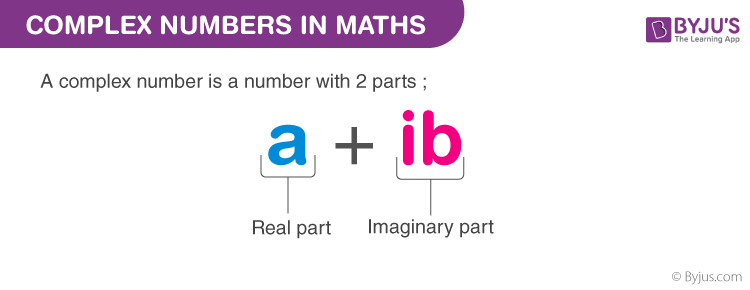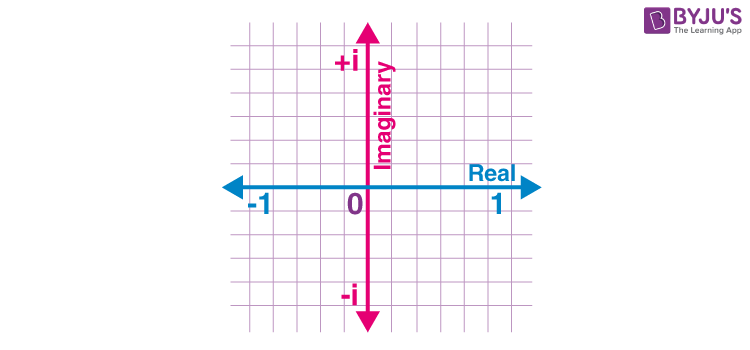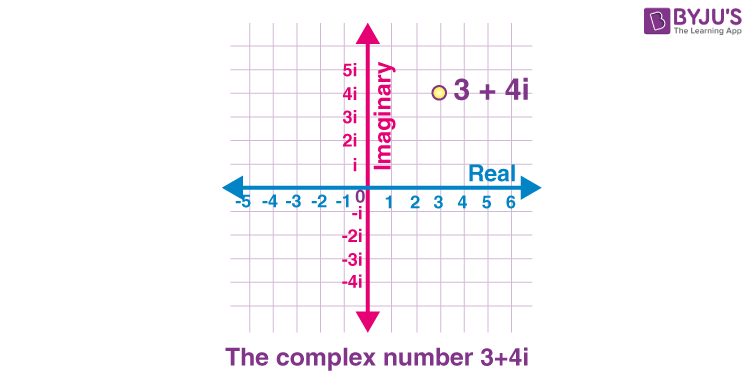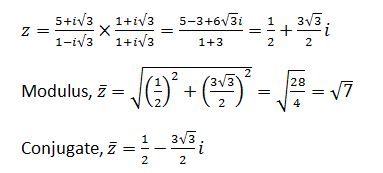# Complex Numbers in Maths

Complex numbers are the numbers that are expressed in the form of a+ib where, a,b are real numbers and  ‘i’ is an imaginary number called “iota”. The value of i = (√-1). For example, 2+3i is a complex number, where 2 is a real number (Re) and 3i is an imaginary number (Im).

 Combination of both the real number and imaginary number is a complex number.

Examples of complex numbers:

• 1 + j
• -13 – 3i
• 0.89 + 1.2 i
• √5 + √2i

An imaginary number is usually represented by ‘i’ or ‘j’, which is equal to √-1. Therefore, the square of the imaginary number gives a negative value.

 Since, i = √-1, so, i2 = -1

The main application of these numbers is to represent periodic motions such as water waves, alternating current, light waves, etc., which rely on sine or cosine waves, etc.

## Definition

The complex number is basically the combination of a real number and an imaginary number. The complex number is in the form of a+ib, where a = real number and ib = imaginary number. Also, a,b belongs to real numbers and i = √-1

Hence, a complex number is a simple representation of addition of two numbers, i.e., real number and an imaginary number. One part of it is purely real and the other part is purely imaginary.### What are Real Numbers?

Any number which is present in a number system such as positive, negative, zero, integer, rational, irrational, fractions, etc. are real numbers. It is represented as Re(). For example: 12, -45, 0, 1/7, 2.8, √5, etc., are all real numbers.

### What are Imaginary Numbers?

The numbers which are not real are imaginary numbers. When we square an imaginary number, it gives a negative result. It is represented as Im(). Example: √-2, √-7, √-11 are all imaginary numbers.

The complex numbers were introduced to solve the equation x2+1 = 0. The roots of the equation are of form x = ±√-1 and no real roots exist. Thus, with the introduction of complex numbers, we have Imaginary roots.

We denote √-1 with the symbol ‘i’, which denotes Iota (Imaginary number).

## Notation

An equation of the form z= a+ib, where a and b are real numbers, is defined to be a complex number. The real part is denoted by Re z = a and the imaginary part is denoted by Im z = ib.

 Z = a + i b

### Examples

See the table below to differentiate between a real number and an imaginary number.

 Complex Number Real Number Imaginary Number -1+2i -1 2i 7-9i 7 -9i -6i 0 -6i (Purely Imaginary) 6 6 0i (Purely Real)

### Is 0 a complex Number?

As we know, 0 is a real number. And real numbers are part of complex numbers. Therefore, 0 is also a complex number and can be represented as 0+0i.

## Graphical representation

In the graph below, check the representation of complex numbers along the axes. Here we can see, the x-axis represents real part and y represents the imaginary part.Let us see an example here. If we have to plot a graph of complex number 3 + 4i, then:## Absolute Value

The absolute value of a real number is the number itself. The absolute value of x is represented by modulus, i.e. |x|. Hence, the modulus of any value always gives a positive value, such that;

|3| = 3

|-3| = 3

Now, in case of complex numbers, finding the modulus has a different method.

Suppose, z = x+iy is a complex number. Then, mod of z, will be:

|z| = √(x2+y2)

This expression is obtained when we apply the Pythagorean theorem in a complex plane. Hence, mod of complex number, z is extended from 0 to z and mod of real numbers x and y is extended from 0 to x and 0 to y respectively. Now these values form a right triangle, where 0 is the vertex of the acute angle. Now, applying Pythagoras theorem,

|z|2 = |x|2+|y|2

|z|2 = x2 + y2

|z| = √(x2+y2)

## Algebraic Operations on Complex Numbers

There can be four types of algebraic operation on complex numbers which are mentioned below. Visit the linked article to know more about these algebraic operations along with solved examples. The four operations on the complex numbers include:

• Subtraction
• Multiplication
• Division

## Roots of Complex Numbers

When we solve a quadratic equation in the form of ax2 +bx+c = 0, the roots of the equations can be determined in three forms;

• Two Distinct Real Roots
• Similar Root
• No Real roots (Complex Roots)

## Complex Number Formulas

While performing the arithmetic operations of complex numbers such as addition and subtraction, combine similar terms. It means that combine the real number with the real number and imaginary number with the imaginary number.

(a + ib) + (c + id) = (a + c) + i(b + d)

### Subtraction

(a + ib) – (c + id) = (a – c) + i(b – d)

### Multiplication

When two complex numbers are multiplied by each other, the multiplication process should be similar to the multiplication of two binomials. It means that the FOIL method (Distributive multiplication process) is used.

(a + ib). (c + id) = (ac – bd) + i(ad + bc)

### Division

The division of two complex numbers can be performed by multiplying the numerator and denominator by its conjugate value of the denominator, and then applying the FOIL Method.

(a + ib) / (c + id) = (ac+bd)/ (c2 + d2) + i(bc – ad) / (c2 + d2)

## Power of Iota (i)

Depending upon the power of “i”, it can take the following values;

i4k+1 = i.i4k+2 = -1 i4k+3 =  -i.i4k = 1

Where k can have an integral value (positive or negative).

Similarly, we can find for the negative power of i, which are as follows;

i-1 = 1 / i

Multiplying and dividing the above term with i, we have;

i-1 = 1 / i  ×  i/i  × i-1  = i / i2 = i / -1 = -i / -1 = -i

Note: √-1 × √-1 = √(-1  × -1) = √1 = 1 contradicts to the fact that i2 = -1.

Therefore, for an imaginary number, √a × √b is not equal to √ab.

## Identities

Let us see some of the identities.

1. (z+ z2)2 = (z1)+ (z2)2 + 2 z1 × z2
2. (z1 – z2)2 = (z1)+ (z2)2 – 2 z1 × z2
3. (z1)2 – (z2)2 =  (z+ z2)(z1 – z2)
4. (z+ z2)3 = (z1)3 + 3(z1)2 z +3(z2)2 z1 + (z2)3
5. (z– z2)3 = (z1)3 – 3(z1)2 z +3(z2)2 z1 – (z2)3

## Properties

The properties of complex numbers are listed below:

• The addition of two conjugate complex numbers will result in a real number
• The multiplication of two conjugate complex number will also result in a real number
• If x and y are the real numbers and x+yi =0, then x =0 and y =0
• If p, q, r, and s are the real numbers and p+qi = r+si, then p = r, and q=s
• The complex number obeys the commutative law of addition and multiplication.

z1+z2  = z2+z1

z1. z2  = z2. z1

• The complex number obeys the associative law of addition and multiplication.

(z1+z2) +z3 = z1 + (z2+z3)

(z1.z2).z3 = z1.(z2.z3)

• The complex number obeys the distributive law

z1.(z2+z3) = z1.z2 + z1.z3

• If the sum of two complex number is real, and also the product of two complex number is also real, then these complex numbers are conjugate to each other.
• For any two complex numbers, say z1 and z2, then |z1+z2| ≤ |z1|+|z2|
• The result of the multiplication of two complex numbers and its conjugate value should result in a complex number and it should be a positive value.

## Modulus and Conjugate

Let z = a+ib be a complex number.

The Modulus of z is represented by |z|.

Mathematically,

$$\begin{array}{l}\left | z \right |= \sqrt{a^{2}+b^{2}}\end{array}$$
$$\begin{array}{l} The\ conjugate\ of\ z\ is\ denoted\ by\ \bar{z}.\end{array}$$

Mathematically,

$$\begin{array}{l}\bar{z}= a – ib \end{array}$$

## Argand Plane and Polar Form

Similar to the XY plane, the Argand(or complex) plane is a system of rectangular coordinates in which the complex number a+ib is represented by the point whose coordinates are a and b.

We find the real and complex components in terms of r and θ, where r is the length of the vector and θ is the angle made with the real axis. Check out the detailed argand plane and polar representation of complex numbers in this article and understand this concept in a detailed way along with solved examples.

## Solved Problems

Example 1: Simplify

a) 16i + 10i(3-i)

b) (7i)(5i)

c) 11i + 13i – 2i

Solution:

a) 16i + 10i(3-i)

= 16i + 10i(3) + 10i (-i)

= 16i +30i – 10 i2

= 46 i – 10 (-1)

= 46i + 10

b) (7i)(5i) = 35  i2 = 35 (-1) = -35

c) 11i + 13i – 2i = 22i

Example 2: Express the following in a+ib form:

(5+√3i)/(1-√3i).

Solution:

Given: (5+√3i)/(1-√3i)## Practice Questions

Based on the explanation given above in this article, try to solve the following questions.

1. Solve (4i-7)+(1-i)
2. Solve i.(i-1)
3. (1+i)/(1-i) =
4. i2000 =
5. Find z2, if z = 6i

## Frequently Asked Questions on Complex Numbers

Q1

### What is meant by complex numbers?

The complex number is the combination of a real number and imaginary number. An example of a complex number is 4+3i. Here 4 is a real number and 3i is an imaginary number.

Q2

### How to divide the complex numbers?

To divide the complex number, multiply the numerator and the denominator by its conjugate. The conjugate of the complex number can be found by changing the sign between the two terms in the denominator value. Then apply the FOIL method to simplify the expression.

Q3

### Mention the arithmetic rules for complex numbers.

The arithmetic rules of complex numbers are:
Addition Rule: (a+bi) + (c+di) = (a+c)+ (b+d)i
Subtraction Rule: (a+bi) – (c+di) = (a-c)+ (b-d)i
Multiplication Rule: (a+bi) . (c+di) = (ac-bd)+(ad+bc)i

Q4

### Write down the additive identity and inverse of complex numbers.

The additive identity of complex numbers is written as (x+yi) + (0+0i) = x+yi. Hence, the additive identity is 0+0i.
The additive inverse of complex numbers is written as (x+yi)+ (-x-yi) = (0+0i). Hence, the additive inverse is -x-yi.

Q5

### Write down the multiplicative identity and inverse of the complex number.

The multiplicative identity of complex numbers is defined as (x+yi). (1+0i) = x+yi. Hence, the multiplicative identity is 1+0i.
The multiplicative identity of complex numbers is defined as (x+yi). (1/x+yi) = 1+0i. Hence, the multiplicative identity is 1/x+yi.

Learn more about the Identities, conjugate of the complex number, and other complex numbers related concepts at BYJU’S. Also, get additional study materials for various maths topics along with practice questions, examples, and tips to be able to learn maths more effectively.

Test your knowledge on Complex Numbers